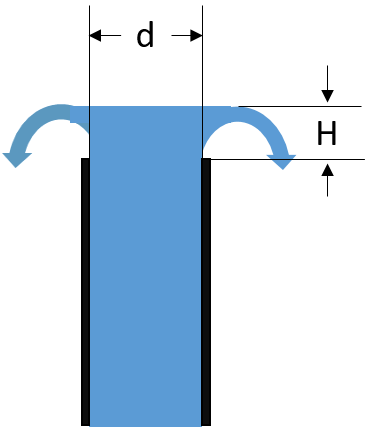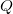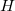# Flow from the End of a Vertical Pipe

This estimates the flow rate out of the end of a vertical pipe. This is not very accurate (+ or - 15%) but can serve as an estimate. Assumes the pipe is vertical and cut off square. Learn more about the units used on this page.Vertical Pipe Diameter:
Height of Free Water Surface Above the end of the Pipe:
Flow Rate:

## The Equations

This calculator usesfor H less than 0.37d andfor H greater than 1.4d, and whichever of the two calculated Qs is less for H in between 0.37d and 1.4d (where these estimates are the least reliable). Reference: U.S. Bureau of Rec. Water Measurement Manual.

and:= Flow rate (gal/min)= Inside diameter of the pipe (inches) (day)= Height of free water surface above the end of the pipe (inches)

WSU Prosser - IAREC, 24106 N Bunn Rd, Prosser WA 99350-8694, 509-786-2226. Contact Us